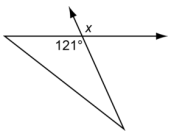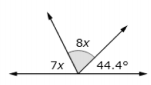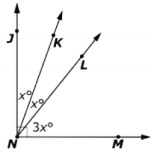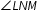# MAFS.7.G.2.5Archived StandardExport Print
Use facts about supplementary, complementary, vertical, and adjacent angles in a multi-step problem to write and solve simple equations for an unknown angle in a figure.
General Information
Subject Area: Mathematics
Domain-Subdomain: Geometry
Cluster: Level 2: Basic Application of Skills & Concepts
Cluster: Solve real-life and mathematical problems involving angle measure, area, surface area, and volume. (Additional Cluster) -

Clusters should not be sorted from Major to Supporting and then taught in that order. To do so would strip the coherence of the mathematical ideas and miss the opportunity to enhance the major work of the grade with the supporting clusters.

Date of Last Rating: 02/14
Status: State Board Approved - Archived
Assessed: Yes
Test Item Specifications

• Assessment Limits :

Items should use angles measured in degrees only.

• Calculator :

Yes

• Context :

Allowable

Sample Test Items (3)
• Test Item #: Sample Item 1
• Question:

A figure is shown.What is the measure, in degrees, of angle x?

• Difficulty: N/A
• Type: EE: Equation Editor

• Test Item #: Sample Item 2
• Question:

A figure is shown.What is the measure, in degrees, of the highlighted angle?

• Difficulty: N/A
• Type: EE: Equation Editor

• Test Item #: Sample Item 3
• Question:

A diagram is shown, with angles labeled in degrees (º).Complete the sentences about the diagram.

A. The equation ___________ can be used to solve for x.

B. The measure of, in degrees, is _____.

• Difficulty: N/A
• Type: EE: Equation Editor

## Related Courses

This benchmark is part of these courses.
1205040: M/J Grade 7 Mathematics (Specifically in versions: 2014 - 2015, 2015 - 2022, 2022 and beyond (current))
1205050: M/J Accelerated Mathematics Grade 7 (Specifically in versions: 2014 - 2015, 2015 - 2020, 2020 - 2022, 2022 and beyond (current))
1204000: M/J Foundational Skills in Mathematics 6-8 (Specifically in versions: 2014 - 2015, 2015 - 2022, 2022 and beyond (current))
7812020: Access M/J Grade 7 Mathematics (Specifically in versions: 2014 - 2015, 2015 - 2018, 2018 - 2019, 2019 - 2022, 2022 and beyond (current))
0101060: M/J Three-Dimensional Studio Art 3 (Specifically in versions: 2014 - 2015, 2015 - 2022, 2022 and beyond (current))

## Related Access Points

Alternate version of this benchmark for students with significant cognitive disabilities.

## Related Resources

Vetted resources educators can use to teach the concepts and skills in this benchmark.

## Educational Game

Tangled Web: An Angle Relationships Game:

You are a robotic spider tangled up in an angular web. Use your knowledge of angle relationships to collect flies and teleport through wormholes to rescue your spider family!

Type: Educational Game

## Educational Software / Tool

Glossary:

This resource is an online glossary to find the meaning of math terms. Students can also use the online glossary to find words that are related to the word typed in the search box. For example: Type in "transversal" and 11 other terms will come up. Click on one of those terms and its meaning is displayed.

Type: Educational Software / Tool

## Formative Assessments

Students are asked use knowledge of angle relationships to write and solve an equation to determine an unknown angle measure.

Type: Formative Assessment

Straight Angles:

Students are asked to write and solve equations to determine unknown angle measures in supplementary angle relationships.

Type: Formative Assessment

Solve for the Angle:

Students are asked to write and solve equations to determine unknown angle measures in supplementary and complementary angle pairs.

Type: Formative Assessment

Find the Angle Measure:

Students are asked to use knowledge of angle relationships to write and solve equations to determine unknown angle measures.

Type: Formative Assessment

Applying Angle Theorems:

This lesson unit is intended to help you assess how well students are able to use geometric properties to solve problems. In particular, it will support you in identifying and helping students who have the following difficulties with solving problems related to the interior or exterior angles of polygons.

Type: Formative Assessment

## Image/Photograph

Angles (Clipart ETC):

This large collection of clipart contains images of angles that can be freely used in lesson plans, worksheets, and presentations.

Type: Image/Photograph

## Lesson Plans

Applying Angle Theorems:

This lesson is intended to help you assess how well students are able to use geometric properties to solve problems. In particular, it will support you in identifying and helping students who have the following difficulties:

• Solving problems relating to using the measures of the interior angles of polygons.
• Solving problems relating to using the measures of the exterior angles of polygons.

Type: Lesson Plan

Angles, angles, everywhere!:

This lesson allows students to discover complementary and supplementary angles on their own. Students will measure the degrees for sets of angles. They will then use their knowledge of straight, right, obtuse, and acute angles to make connections to complementary, supplementary, adjacent, and vertical angles. This lesson will complement all different learning styles because it provides kinesthetic, auditory, and visual learning.

Type: Lesson Plan

Kissing Vs:

This lesson uses a paper cutting activity to teach vertical angles. The lesson provides examples in which students must solve equations to find missing measures.

Type: Lesson Plan

Students, through a hands-on-activity and guided practice, will explore parallel lines intersected by a transversal and the measurements and relationships of the 8 angles created. They will solve for missing measurements when given a single angle's measurement. They will also use the relationships between angles to set up equations and solve for a variable.

Type: Lesson Plan

## Original Student Tutorials

Playground Angles Part 1:

Explore complementary and supplementary angles around the playground with Jacob in this interactive tutorial.

This is Part 1 in a two-part series. Click HERE to open Playground Angles: Part 2.

Type: Original Student Tutorial

Playground Angles: Part 2:

Help Jacob write and solve equations to find missing angle measures based on the relationship between angles that sum to 90 degrees and 180 degrees in this playground-themed, interactive tutorial.

This is Part 2 in a two-part series. Click HERE to open Playground Angles: Part 1.

Type: Original Student Tutorial

## Tutorials

Finding the Measure of Complementary Angles:

The video will use algebra to find the measure of two angles whose sum equals 90 degrees, better known as complementary angles.

Type: Tutorial

Find Measure of Complementary Angles:

Watch as we use algebra to find the measure of two complementary angles.

Type: Tutorial

Find Measure of Supplementary Angles:

Watch as we use algebra to find the measure of supplementary angles, whose sum is 180 degrees.

Type: Tutorial

Find Measure of Vertical Angles:

This video uses knowledge of vertical angles to solve for the variable and the angle measures.

Type: Tutorial

Introduction to Vertical Angles:

This video uses facts about supplementary and adjacent angles to introduce vertical angles.

Type: Tutorial

Find Measure of Angles in a Word Problem:

This video demonstrates solving a word problem involving angle measures.

Type: Tutorial

Complementary and Supplementary Angles:

The video will demonstrate the difference between supplementary angles and complementary angles, by using the given measurements of angles.

Type: Tutorial

Proof: Vertical Angles are Equal:

This 5 minute video gives the proof that vertical angles are equal.

Type: Tutorial

Vertical, Adjacent and Linearly Paired Angles:

This resource will allow students to have a good understanding about vertical, adjacent and linear pairs of angles.

Type: Tutorial

## MFAS Formative Assessments

Find the Angle Measure:

Students are asked to use knowledge of angle relationships to write and solve equations to determine unknown angle measures.

Solve for the Angle:

Students are asked to write and solve equations to determine unknown angle measures in supplementary and complementary angle pairs.

Straight Angles:

Students are asked to write and solve equations to determine unknown angle measures in supplementary angle relationships.

Students are asked use knowledge of angle relationships to write and solve an equation to determine an unknown angle measure.

## Original Student Tutorials Mathematics - Grades 6-8

Playground Angles Part 1:

Explore complementary and supplementary angles around the playground with Jacob in this interactive tutorial.

This is Part 1 in a two-part series. Click HERE to open Playground Angles: Part 2.

Playground Angles: Part 2:

Help Jacob write and solve equations to find missing angle measures based on the relationship between angles that sum to 90 degrees and 180 degrees in this playground-themed, interactive tutorial.

This is Part 2 in a two-part series. Click HERE to open Playground Angles: Part 1.

## Student Resources

Vetted resources students can use to learn the concepts and skills in this benchmark.

## Original Student Tutorials

Playground Angles Part 1:

Explore complementary and supplementary angles around the playground with Jacob in this interactive tutorial.

This is Part 1 in a two-part series. Click HERE to open Playground Angles: Part 2.

Type: Original Student Tutorial

Playground Angles: Part 2:

Help Jacob write and solve equations to find missing angle measures based on the relationship between angles that sum to 90 degrees and 180 degrees in this playground-themed, interactive tutorial.

This is Part 2 in a two-part series. Click HERE to open Playground Angles: Part 1.

Type: Original Student Tutorial

## Educational Software / Tool

Glossary:

This resource is an online glossary to find the meaning of math terms. Students can also use the online glossary to find words that are related to the word typed in the search box. For example: Type in "transversal" and 11 other terms will come up. Click on one of those terms and its meaning is displayed.

Type: Educational Software / Tool

## Tutorials

Finding the Measure of Complementary Angles:

The video will use algebra to find the measure of two angles whose sum equals 90 degrees, better known as complementary angles.

Type: Tutorial

Find Measure of Complementary Angles:

Watch as we use algebra to find the measure of two complementary angles.

Type: Tutorial

Find Measure of Supplementary Angles:

Watch as we use algebra to find the measure of supplementary angles, whose sum is 180 degrees.

Type: Tutorial

Find Measure of Vertical Angles:

This video uses knowledge of vertical angles to solve for the variable and the angle measures.

Type: Tutorial

Introduction to Vertical Angles:

This video uses facts about supplementary and adjacent angles to introduce vertical angles.

Type: Tutorial

Find Measure of Angles in a Word Problem:

This video demonstrates solving a word problem involving angle measures.

Type: Tutorial

Complementary and Supplementary Angles:

The video will demonstrate the difference between supplementary angles and complementary angles, by using the given measurements of angles.

Type: Tutorial

Proof: Vertical Angles are Equal:

This 5 minute video gives the proof that vertical angles are equal.

Type: Tutorial

Vertical, Adjacent and Linearly Paired Angles:

This resource will allow students to have a good understanding about vertical, adjacent and linear pairs of angles.

Type: Tutorial

## Parent Resources

Vetted resources caregivers can use to help students learn the concepts and skills in this benchmark.

## Educational Software / Tool

Glossary:

This resource is an online glossary to find the meaning of math terms. Students can also use the online glossary to find words that are related to the word typed in the search box. For example: Type in "transversal" and 11 other terms will come up. Click on one of those terms and its meaning is displayed.

Type: Educational Software / Tool